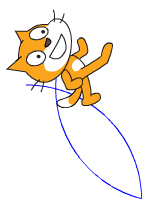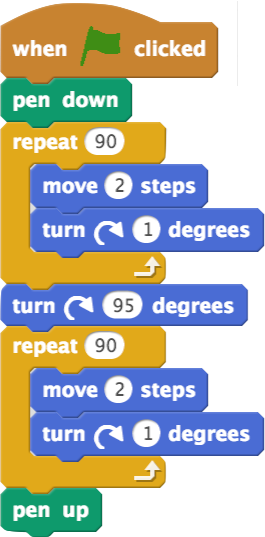Select Page

## Debugging Skills

### Homework 4, Part 2: Drawing Petals

For my computer science course, I was given an assignment to draw the following shape by pair programming with my friend from class.

## Prompt:

Draw a set of petals.
Try to draw the picture below.
Hint: Start each petal at the center of the flower.The first thing we wanted to do is create a script that will clear the screen, because this makes debugging easier! So we combined blocks to create this script.Now that we have taken care of housekeeping, it’s time to tackle the real problem. How can we draw these petals? We realized that each petal looks exactly like the other petals! We chose to start by making one and later we can use a “repeat” block to make the other ones.

We decided to draw one petal. We created a line from each pointed edge, splitting the shape in half.We discovered that half of a petal looks a lot like part of a circl – 1/4 of a circle, to be precise. We know a full circle is 360 degrees and that a 1/4 of a circle involves turning 90 degrees, so we made the sprite move one step and turn 1 degree 90 times.We were super excited! We made half of a petal, but it was really small.Which part of the script above do we need to change to make the petal bigger?

We decided to have the cat move 2 steps for every angle so the petals will be giant!

Now we had to figure out how much the cat needs to turn to finish this petal. But we didn’t know what to do! I remembered that guessing and checking is a really good debugging strategy to figure out how much to turn the cat and suggested we try it.

Fill in the blanks and circle whether the cat need to turn more or less for the following guesses.

First we guessed 45 degrees.Do we need to turn more or less?

Here is our next guess:How many degrees did we guess?

Do we need to turn more or less?

That still wasn’t enough! Here is our next guess:How many degrees did we guess?

Do we need to turn more or less?

Guessing and checking is working! But we still aren’t quite there yet. Here is our next guess:How many degrees did we guess?

Do we need to turn more or less?

I was so excited we were getting closer! Here is our next guess:How many degrees did we guess?

Do we need to turn more or less?

We could tell how close we were! Here is our next guess:How many degrees did we guess?

Do we need to turn more or less?

We were almost there. Here is our last guess:We found it! The correct angle for the sprite to turn is 90 degrees.

We also found out that if you look really close, you can see that the angle of the corner of the petal is 90 degrees!How many degrees does the sprite turn in total while drawing 1 petal?

Explain what each of these parts of the script does using English. For example, if you were describing a script that draws a star, you should say “Draws a star” not “Moves 5 steps, turns 72 degrees…”What do lines 3-5 do?

What does line 6 do?

What do lines 7-9 do?

Now we needed to repeat this shape more, a total of 4 repeats, to make all four petals. We decided to put a repeat block in the script.

Which of the blocks above would you put a repeat around to make the script draw four petals? Check all that apply.

We discovered that, because the sprite finishes drawing each petal facing a different direction along the x and the y axis, we only needed to add a repeat block to make the correct shape! So, we created the final script below.The picture below shows the final flower drawing. Some of the sides are labeled with numbers that tell the order they were drawn in.What number side is side A?

What number side is side B?